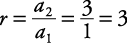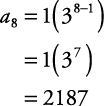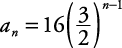## Geometric Sequence

A geometric sequence is a sequence in which each term is found by multiplying the preceding term by the same value. Its general term is

a n = a 1 r n – 1

The value r is called the common ratio. It is found by taking any term in the sequence and dividing it by its preceding term.

##### Example 1

Find the common ratio in each of the following geometric sequences. Then express each sequence in the form a n = a 1 r n – 1 and find the eighth term of the sequence.

1. 1, 3, 9, 27, …

2. 64, –16, 4, –1, …

3. 16, 24, 36, 54, …

1. 1, 3, 9, 27, …

SinceThen a n = 1(3 n–1 )

Therefore, the eighth term of the sequence is2. 64, –16, 4, –1, …

SinceThenTherefore, the eighth term of the sequence is3. 16, 24, 36, 54, …

SinceThenTherefore, the eighth term of the sequence is